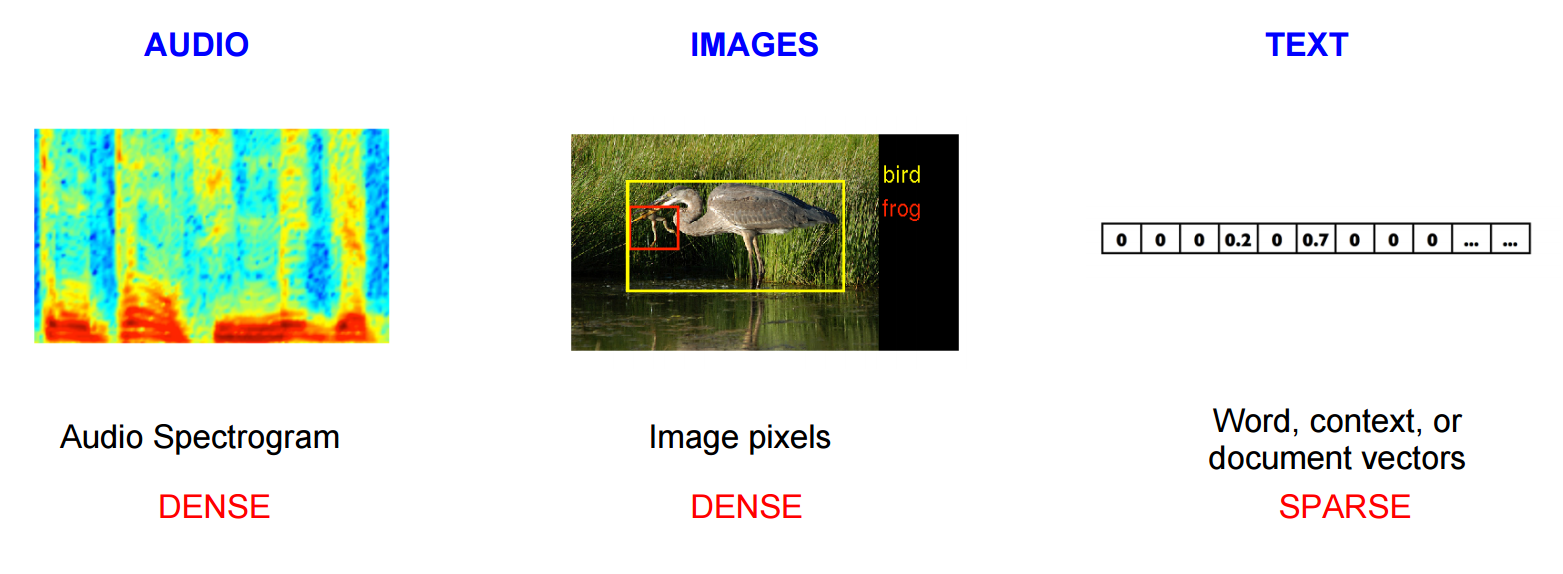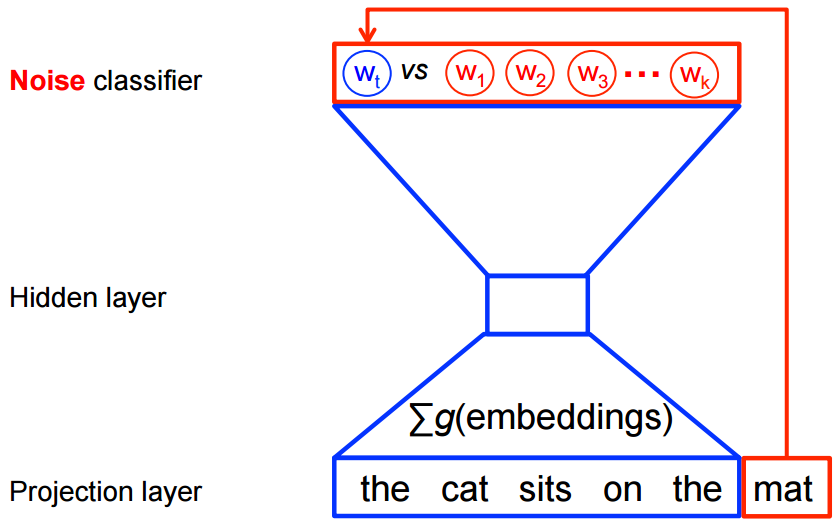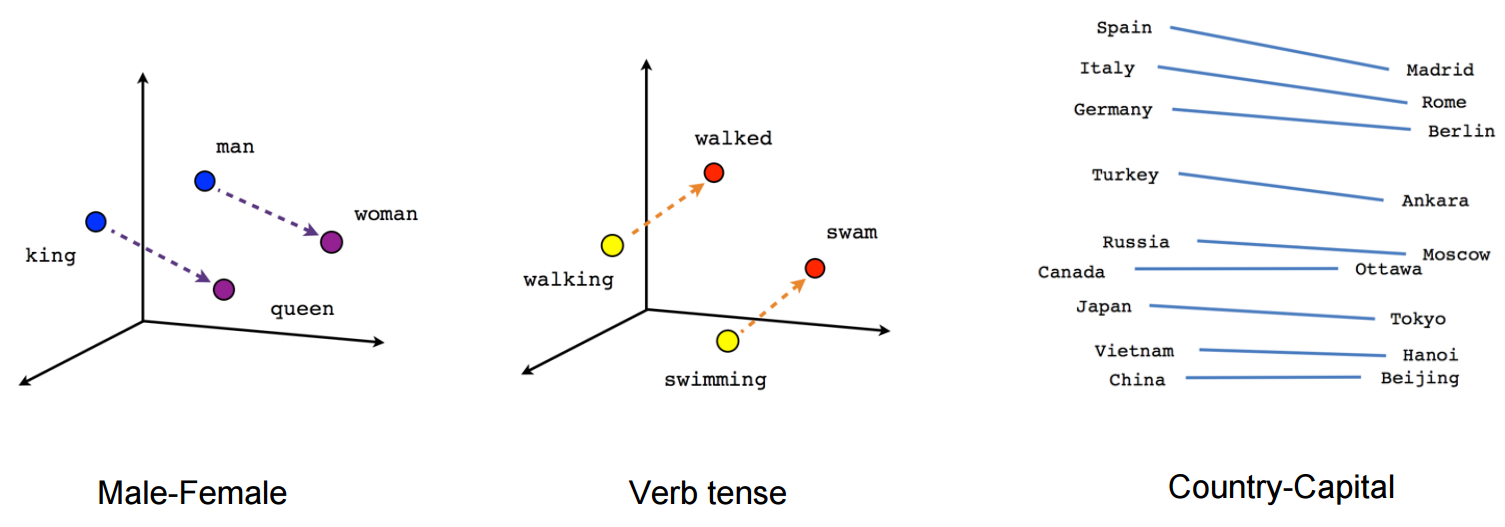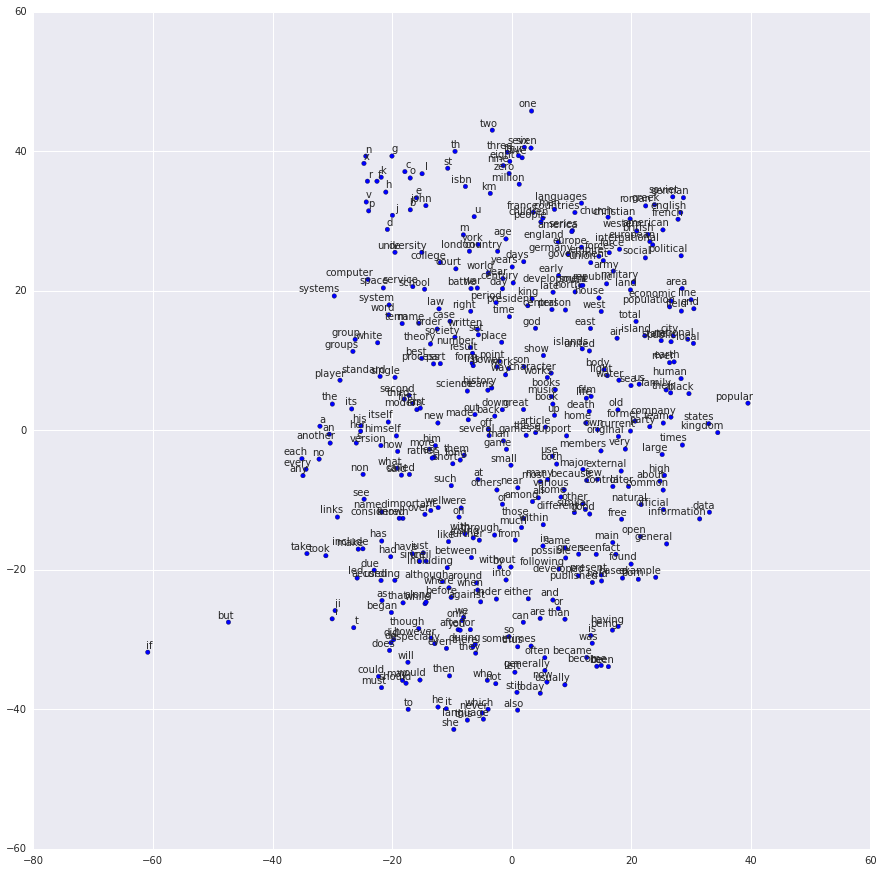TensorFlow 2.0 Beta is available

# Vector Representations of Words

In this tutorial we look at the word2vec model by Mikolov et al. This model is used for learning vector representations of words, called "word embeddings".

## Highlights

This tutorial is meant to highlight the interesting, substantive parts of building a word2vec model in TensorFlow.

• We start by giving the motivation for why we would want to represent words as vectors.
• We look at the intuition behind the model and how it is trained (with a splash of math for good measure).
• We also show a simple implementation of the model in TensorFlow.
• Finally, we look at ways to make the naive version scale better.

We walk through the code later during the tutorial, but if you'd prefer to dive straight in, feel free to look at the minimalistic implementation in tensorflow/examples/tutorials/word2vec/word2vec_basic.py This basic example contains the code needed to download some data, train on it a bit and visualize the result. Once you get comfortable with reading and running the basic version, you can graduate to models/tutorials/embedding/word2vec.py which is a more serious implementation that showcases some more advanced TensorFlow principles about how to efficiently use threads to move data into a text model, how to checkpoint during training, etc.

But first, let's look at why we would want to learn word embeddings in the first place. Feel free to skip this section if you're an Embedding Pro and you'd just like to get your hands dirty with the details.

## Motivation: Why learn word embeddings?

Image and audio processing systems work with rich, high-dimensional datasets encoded as vectors of the individual raw pixel-intensities for image data, or e.g. power spectral density coefficients for audio data. For tasks like object or speech recognition we know that all the information required to successfully perform the task is encoded in the data (because humans can perform these tasks from the raw data). However, natural language processing systems traditionally treat words as discrete atomic symbols, and therefore 'cat' may be represented as Id537 and 'dog' as Id143. These encodings are arbitrary, and provide no useful information to the system regarding the relationships that may exist between the individual symbols. This means that the model can leverage very little of what it has learned about 'cats' when it is processing data about 'dogs' (such that they are both animals, four-legged, pets, etc.). Representing words as unique, discrete ids furthermore leads to data sparsity, and usually means that we may need more data in order to successfully train statistical models. Using vector representations can overcome some of these obstacles.Vector space models (VSMs) represent (embed) words in a continuous vector space where semantically similar words are mapped to nearby points ('are embedded nearby each other'). VSMs have a long, rich history in NLP, but all methods depend in some way or another on the Distributional Hypothesis, which states that words that appear in the same contexts share semantic meaning. The different approaches that leverage this principle can be divided into two categories: count-based methods (e.g. Latent Semantic Analysis), and predictive methods (e.g. neural probabilistic language models).

This distinction is elaborated in much more detail by Baroni et al., but in a nutshell: Count-based methods compute the statistics of how often some word co-occurs with its neighbor words in a large text corpus, and then map these count-statistics down to a small, dense vector for each word. Predictive models directly try to predict a word from its neighbors in terms of learned small, dense embedding vectors (considered parameters of the model).

Word2vec is a particularly computationally-efficient predictive model for learning word embeddings from raw text. It comes in two flavors, the Continuous Bag-of-Words model (CBOW) and the Skip-Gram model (Section 3.1 and 3.2 in Mikolov et al.). Algorithmically, these models are similar, except that CBOW predicts target words (e.g. 'mat') from source context words ('the cat sits on the'), while the skip-gram does the inverse and predicts source context-words from the target words. This inversion might seem like an arbitrary choice, but statistically it has the effect that CBOW smoothes over a lot of the distributional information (by treating an entire context as one observation). For the most part, this turns out to be a useful thing for smaller datasets. However, skip-gram treats each context-target pair as a new observation, and this tends to do better when we have larger datasets. We will focus on the skip-gram model in the rest of this tutorial.

## Scaling up with noise-contrastive training

Neural probabilistic language models are traditionally trained using the maximum likelihood (ML) principle to maximize the probability of the next word $$w_t$$ (for "target") given the previous words $$h$$ (for "history") in terms of a softmax function,

\begin{align} P(w_t | h) &= \text{softmax} (\text{score} (w_t, h)) \\ &= \frac{\exp \{ \text{score} (w_t, h) \} } {\sum_\text{Word w' in Vocab} \exp \{ \text{score} (w', h) \} } \end{align}

where $$\text{score} (w_t, h)$$ computes the compatibility of word $$w_t$$ with the context $$h$$ (a dot product is commonly used). We train this model by maximizing its log-likelihood on the training set, i.e. by maximizing

\begin{align} J_\text{ML} &= \log P(w_t | h) \\ &= \text{score} (w_t, h) - \log \left( \sum_\text{Word w' in Vocab} \exp \{ \text{score} (w', h) \} \right). \end{align}

This yields a properly normalized probabilistic model for language modeling. However this is very expensive, because we need to compute and normalize each probability using the score for all other $$V$$ words $$w'$$ in the current context $$h$$, at every training step.On the other hand, for feature learning in word2vec we do not need a full probabilistic model. The CBOW and skip-gram models are instead trained using a binary classification objective (logistic regression) to discriminate the real target words $$w_t$$ from $$k$$ imaginary (noise) words $$\tilde w$$, in the same context. We illustrate this below for a CBOW model. For skip-gram the direction is simply inverted.Mathematically, the objective (for each example) is to maximize

$$J_\text{NEG} = \log Q_\theta(D=1 |w_t, h) + k \mathop{\mathbb{E}}_{\tilde w \sim P_\text{noise}} \left[ \log Q_\theta(D = 0 |\tilde w, h) \right]$$

where $$Q_\theta(D=1 | w, h)$$ is the binary logistic regression probability under the model of seeing the word $$w$$ in the context $$h$$ in the dataset $$D$$, calculated in terms of the learned embedding vectors $$\theta$$. In practice we approximate the expectation by drawing $$k$$ contrastive words from the noise distribution (i.e. we compute a Monte Carlo average).

This objective is maximized when the model assigns high probabilities to the real words, and low probabilities to noise words. Technically, this is called Negative Sampling, and there is good mathematical motivation for using this loss function: The updates it proposes approximate the updates of the softmax function in the limit. But computationally it is especially appealing because computing the loss function now scales only with the number of noise words that we select ($$k$$), and not all words in the vocabulary ($$V$$). This makes it much faster to train. We will actually make use of the very similar noise-contrastive estimation (NCE) loss, for which TensorFlow has a handy helper function tf.nn.nce_loss().

Let's get an intuitive feel for how this would work in practice!

## The skip-gram model

As an example, let's consider the dataset

the quick brown fox jumped over the lazy dog

We first form a dataset of words and the contexts in which they appear. We could define 'context' in any way that makes sense, and in fact people have looked at syntactic contexts (i.e. the syntactic dependents of the current target word, see e.g. Levy et al.), words-to-the-left of the target, words-to-the-right of the target, etc. For now, let's stick to the vanilla definition and define 'context' as the window of words to the left and to the right of a target word. Using a window size of 1, we then have the dataset

([the, brown], quick), ([quick, fox], brown), ([brown, jumped], fox), ...

of (context, target) pairs. Recall that skip-gram inverts contexts and targets, and tries to predict each context word from its target word, so the task becomes to predict 'the' and 'brown' from 'quick', 'quick' and 'fox' from 'brown', etc. Therefore our dataset becomes

(quick, the), (quick, brown), (brown, quick), (brown, fox), ...

of (input, output) pairs. The objective function is defined over the entire dataset, but we typically optimize this with stochastic gradient descent (SGD) using one example at a time (or a 'minibatch' of batch_size examples, where typically 16 <= batch_size <= 512). So let's look at one step of this process.

Let's imagine at training step $$t$$ we observe the first training case above, where the goal is to predict the from quick. We select num_noise number of noisy (contrastive) examples by drawing from some noise distribution, typically the unigram distribution, $$P(w)$$. For simplicity let's say num_noise=1 and we select sheep as a noisy example. Next we compute the loss for this pair of observed and noisy examples, i.e. the objective at time step $$t$$ becomes

$$J^{(t)}_\text{NEG} = \log Q_\theta(D=1 | \text{the, quick}) + \log(Q_\theta(D=0 | \text{sheep, quick}))$$

The goal is to make an update to the embedding parameters $$\theta$$ to improve (in this case, maximize) this objective function. We do this by deriving the gradient of the loss with respect to the embedding parameters $$\theta$$, i.e. $$\frac{\partial}{\partial \theta} J_\text{NEG}$$ (luckily TensorFlow provides easy helper functions for doing this!). We then perform an update to the embeddings by taking a small step in the direction of the gradient. When this process is repeated over the entire training set, this has the effect of 'moving' the embedding vectors around for each word until the model is successful at discriminating real words from noise words.

We can visualize the learned vectors by projecting them down to 2 dimensions using for instance something like the t-SNE dimensionality reduction technique. When we inspect these visualizations it becomes apparent that the vectors capture some general, and in fact quite useful, semantic information about words and their relationships to one another. It was very interesting when we first discovered that certain directions in the induced vector space specialize towards certain semantic relationships, e.g. male-female, verb tense and even country-capital relationships between words, as illustrated in the figure below (see also for example Mikolov et al., 2013).This explains why these vectors are also useful as features for many canonical NLP prediction tasks, such as part-of-speech tagging or named entity recognition (see for example the original work by Collobert et al., 2011 (pdf), or follow-up work by Turian et al., 2010).

But for now, let's just use them to draw pretty pictures!

## Building the graph

This is all about embeddings, so let's define our embedding matrix. This is just a big random matrix to start. We'll initialize the values to be uniform in the unit cube.

embeddings = tf.Variable(
tf.random_uniform([vocabulary_size, embedding_size], -1.0, 1.0))


The noise-contrastive estimation loss is defined in terms of a logistic regression model. For this, we need to define the weights and biases for each word in the vocabulary (also called the output weights as opposed to the input embeddings). So let's define that.

nce_weights = tf.Variable(
tf.truncated_normal([vocabulary_size, embedding_size],
stddev=1.0 / math.sqrt(embedding_size)))
nce_biases = tf.Variable(tf.zeros([vocabulary_size]))


Now that we have the parameters in place, we can define our skip-gram model graph. For simplicity, let's suppose we've already integerized our text corpus with a vocabulary so that each word is represented as an integer (see tensorflow/examples/tutorials/word2vec/word2vec_basic.py for the details). The skip-gram model takes two inputs. One is a batch full of integers representing the source context words, the other is for the target words. Let's create placeholder nodes for these inputs, so that we can feed in data later.

# Placeholders for inputs
train_inputs = tf.placeholder(tf.int32, shape=[batch_size])
train_labels = tf.placeholder(tf.int32, shape=[batch_size, 1])


Now what we need to do is look up the vector for each of the source words in the batch. TensorFlow has handy helpers that make this easy.

embed = tf.nn.embedding_lookup(embeddings, train_inputs)


Ok, now that we have the embeddings for each word, we'd like to try to predict the target word using the noise-contrastive training objective.

# Compute the NCE loss, using a sample of the negative labels each time.
loss = tf.reduce_mean(
tf.nn.nce_loss(weights=nce_weights,
biases=nce_biases,
labels=train_labels,
inputs=embed,
num_sampled=num_sampled,
num_classes=vocabulary_size))


Now that we have a loss node, we need to add the nodes required to compute gradients and update the parameters, etc. For this we will use stochastic gradient descent, and TensorFlow has handy helpers to make this easy as well.

# We use the SGD optimizer.


## Training the model

Training the model is then as simple as using a feed_dict to push data into the placeholders and calling tf.Session.run with this new data in a loop.

for inputs, labels in generate_batch(...):
feed_dict = {train_inputs: inputs, train_labels: labels}
_, cur_loss = session.run([optimizer, loss], feed_dict=feed_dict)


See the full example code in tensorflow/examples/tutorials/word2vec/word2vec_basic.py.

## Visualizing the learned embeddings

After training has finished we can visualize the learned embeddings using t-SNE.Et voila! As expected, words that are similar end up clustering nearby each other. For a more heavyweight implementation of word2vec that showcases more of the advanced features of TensorFlow, see the implementation in models/tutorials/embedding/word2vec.py.

## Evaluating embeddings: analogical reasoning

Embeddings are useful for a wide variety of prediction tasks in NLP. Short of training a full-blown part-of-speech model or named-entity model, one simple way to evaluate embeddings is to directly use them to predict syntactic and semantic relationships like king is to queen as father is to ?. This is called analogical reasoning and the task was introduced by Mikolov and colleagues . Download the dataset for this task from download.tensorflow.org.

To see how we do this evaluation, have a look at the build_eval_graph() and eval() functions in models/tutorials/embedding/word2vec.py.

The choice of hyperparameters can strongly influence the accuracy on this task. To achieve state-of-the-art performance on this task requires training over a very large dataset, carefully tuning the hyperparameters and making use of tricks like subsampling the data, which is out of the scope of this tutorial.

## Optimizing the implementation

Our vanilla implementation showcases the flexibility of TensorFlow. For example, changing the training objective is as simple as swapping out the call to tf.nn.nce_loss() for an off-the-shelf alternative such as tf.nn.sampled_softmax_loss(). If you have a new idea for a loss function, you can manually write an expression for the new objective in TensorFlow and let the optimizer compute its derivatives. This flexibility is invaluable in the exploratory phase of machine learning model development, where we are trying out several different ideas and iterating quickly.

Once you have a model structure you're satisfied with, it may be worth optimizing your implementation to run more efficiently (and cover more data in less time). For example, the naive code we used in this tutorial would suffer compromised speed because we use Python for reading and feeding data items -- each of which require very little work on the TensorFlow back-end. If you find your model is seriously bottlenecked on input data, you may want to implement a custom data reader for your problem, as described in New Data Formats. For the case of Skip-Gram modeling, we've actually already done this for you as an example in models/tutorials/embedding/word2vec.py.

If your model is no longer I/O bound but you want still more performance, you can take things further by writing your own TensorFlow Ops, as described in Adding a New Op. Again we've provided an example of this for the Skip-Gram case models/tutorials/embedding/word2vec_optimized.py. Feel free to benchmark these against each other to measure performance improvements at each stage.

## Conclusion

In this tutorial we covered the word2vec model, a computationally efficient model for learning word embeddings. We motivated why embeddings are useful, discussed efficient training techniques and showed how to implement all of this in TensorFlow. Overall, we hope that this has show-cased how TensorFlow affords you the flexibility you need for early experimentation, and the control you later need for bespoke optimized implementation.